# EDTA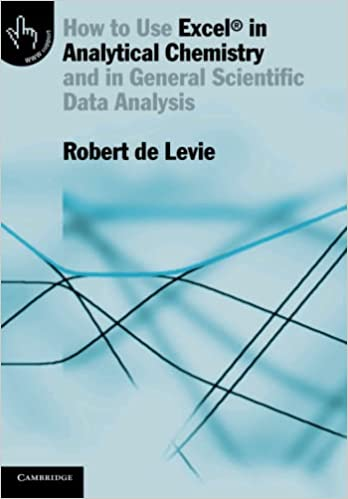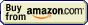## Complexometric titration » End point detection

Indicators used in complexometric titration are to some extent similar to those used in acid-base titrations. Their color changes depending on the concentration of metal ions, just like color of pH indicators changes depending on the H+ concentration. Mechanism of this color change is different, as all complexometric indicators are just complexing agents, changing their color depending on whether they are free in the solution, or ligands in the complex. In most cases they are also weak acids or bases, and quite often their color depends on the solution pH.

For a metal indicator to be useful, several conditions must be met. First of all - its stability constant must be high enough so that the free metal when present in the solution is easily complexed, but lower than the stability of the complex with titrant. Otherwise indicator will be not replaced in the complex and there will be no color change of the solution. Secondly, both indicator complex creation and dissociation reactions must be fast, so that the equilibrium in the solution is achieved almost immediately after titrant addition.

Let's see how concentration of the free indicator changes in the vicinity of the equivalence point of the calcium titration. Let's assume we are titrating 0.1M calcium with 0.1M EDTA in the presence of murexide. Complexation constants are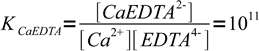1

and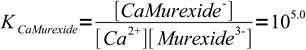2

What we will calculate is a fraction of free murexide, responsible for violet solution color. We will calculate it for both 99.9 and 100.1% titration.

First of all - we have to calculate concentrations of Ca2+ in both cases. That's not difficult - at 99.9% (that is, before the equivalence point) concentration of the metal is just that of what was left: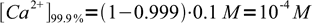3

After the end point concentration is determined by the concentration of complex and concentration of excess ligand. Instead of calculating exact values, we will just use their ratio - we know that at 100.1% we have added 0.1% excess of titrant, so ratio of complex to free ligand concentration is 100:0.1. Note, that even if we will use calculated concentrations we will get exactly the same value, as volume of the solution will cancel out.4

Initial concentration of murexide (CMurexide) in titrated solution is about 10-5M (estimated from the titration procedure), that's enough to color the solution. From the mass balance we know that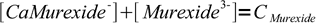5

Solving for complex concentration and substituting complex concentration in the formation constant we get6

or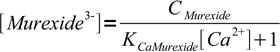7

However, we wanted fraction of the free murexide, so we will divide last formula by CMurexide:8

We are ready now to calculate concentrations of Ca2+ and fractions of free murexide:

%[Ca2+]fmurexide
99.910-40.091
100.110-80.999

As we can see, 0.1% before equivalence point solution color is dominated by the color of calcium murexide complex, 0.1% after equivalence point concentration of this complex is negligible as there is almost only free murexide present.

Example above is heavily simplified, as in fact we should take both dilution and - more importantly - pH into account. Both EDTA and murexide are weak, multiprotic acids, and even at the high pH used during Ca2+ titration they are partially protonated. To account for protonation we should use conditional formation constants in our calculations. However, full calculations, while interesting, will be much more complicated and the final conclusion will be identical, just the numbers will be slightly different.

Probably most popular and universal indicator used in complexometric titrations is Eriochrome Black T. For pH below 6, its color is red, between 7 and 11 - blue, above 12 - yellow-orange. Complexed form is always wine red. Eriochrome Black T solutions are unstable, so it is prepared as a solid, mixed with NaCl (100 mg of indicator ground with 20 g of NaCl), or as a fresh solution (shelf life one day).

Similar in its properties, but much more stable (solutions can be kept for up to a year) is calmagite. It can be used instead of Eriochrome Black T in most titrations.

Other popular indicators are pyrocatechin violet, murexide and PAN. Also sulfosalicylic acid is used, although it differs from other indicators listed, as it is used only for one cation (Fe3+) and is a one color indicator.

In some cases redox indicators can be used. For example when titrating Fe3+ redox potential of the solution is high in the presence of excess Fe3+, which keeps some of the redox indicators in the oxidized form. After equivalence point concentration of Fe3+ becomes very small and indicator gets reduced. Other interesting approach is used in the case of Al3+ titration. Al3+ can't be titrated directly with EDTA, as it reacts too slow. To avoid problems back titration is used - and excess EDTA is titrated with Zn2+. Before titration some small amounts of ferrocyanide and ferricyanide are added to the solution, together with diphenylbenzidine. After equivalence point excess Zn2+ precipitates ferrocyanide increasing redox potential of the solution, and diphenylbenzidine gets oxidized.

Finally, there are specific ways of detecting end point for other types of complexometric titrations. For example in the case of cyanides determination with Ag+ solution (Liebig-Dénigès method) reaction that takes place is

Ag+ + 2CN- → Ag(CN)2-

and after equivalence point, when excess Ag+ is added

Ag+ + Ag(CN)2- → 2AgCN

Solid AgCN makes solution turbid and is easy to spot, so there is no need for any other indicator.